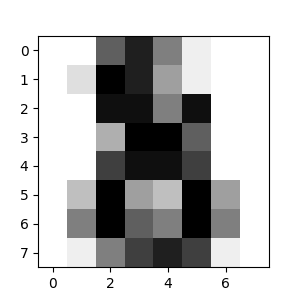# 使用 scikit-learn 介绍机器学习

## 机器学习：问题设置

• 监督学习 , 其中数据带有一个附加属性，即我们想要预测的结果值（ 点击此处 转到 scikit-learn 监督学习页面）。这个问题可以是:

• 分类 : 样本属于两个或更多个类，我们想从已经标记的数据中学习如何预测未标记数据的类别。 分类问题的一个例子是手写数字识别，其目的是将每个输入向量分配给有限数目的离散类别之一。 我们通常把分类视作监督学习的一个离散形式（区别于连续形式），从有限的类别中，给每个样本贴上正确的标签。

• 回归 : 如果期望的输出由一个或多个连续变量组成，则该任务称为 回归 。 回归问题的一个例子是预测鲑鱼的长度是其年龄和体重的函数。

• 无监督学习, 其中训练数据由没有任何相应目标值的一组输入向量x组成。这种问题的目标可能是在数据中发现彼此类似的示例所聚成的组，这种问题称为 聚类 , 或者，确定输入空间内的数据分布，称为 密度估计 ，又或从高维数据投影数据空间缩小到二维或三维以进行 可视化点击此处 转到 scikit-learn 无监督学习页面）。

## 加载示例数据集

scikit-learn 提供了一些标准数据集，例如 用于分类的 irisdigits 数据集 和 波士顿房价回归数据集 .

``````\$ python
>>> from sklearn import datasets

``````

``````>>> print(digits.data)
[[  0.   0.   5. ...,   0.   0.   0.]
[  0.   0.   0. ...,  10.   0.   0.]
[  0.   0.   0. ...,  16.   9.   0.]
...,
[  0.   0.   1. ...,   6.   0.   0.]
[  0.   0.   2. ...,  12.   0.   0.]
[  0.   0.  10. ...,  12.   1.   0.]]

``````

``````>>> digits.target
array([0, 1, 2, ..., 8, 9, 8])

``````

digits.images array([[ 0., 0., 5., 13., 9., 1., 0., 0.], [ 0., 0., 13., 15., 10., 15., 5., 0.], [ 0., 3., 15., 2., 0., 11., 8., 0.], [ 0., 4., 12., 0., 0., 8., 8., 0.], [ 0., 5., 8., 0., 0., 9., 8., 0.], [ 0., 4., 11., 0., 1., 12., 7., 0.], [ 0., 2., 14., 5., 10., 12., 0., 0.], [ 0., 0., 6., 13., 10., 0., 0., 0.]]) ``` 该 数据集上的简单示例 说明了如何从原始数据开始调整，形成可以在 scikit-learn 中使用的数据。

## 学习和预测

``````>>> from sklearn import svm
>>> clf = svm.SVC(gamma=0.001, C=100.)

``````

``````>>> clf.fit(digits.data[:-1], digits.target[:-1])
SVC(C=100.0, cache_size=200, class_weight=None, coef0=0.0,
decision_function_shape='ovr', degree=3, gamma=0.001, kernel='rbf',
max_iter=-1, probability=False, random_state=None, shrinking=True,
tol=0.001, verbose=False)

``````

``````>>> clf.predict(digits.data[-1:])
array()

``````## 模型持久化

``````>>> from sklearn import svm
>>> from sklearn import datasets
>>> clf = svm.SVC()
>>> X, y = iris.data, iris.target
>>> clf.fit(X, y)
SVC(C=1.0, cache_size=200, class_weight=None, coef0=0.0,
decision_function_shape='ovr', degree=3, gamma='auto', kernel='rbf',
max_iter=-1, probability=False, random_state=None, shrinking=True,
tol=0.001, verbose=False)

>>> import pickle
>>> s = pickle.dumps(clf)
>>> clf2.predict(X[0:1])
array()
>>> y
0

``````

``````>>> from joblib import dump, load
>>> dump(clf, 'filename.joblib')
``````

``````>>> clf = load('filename.joblib')

``````

`joblib.dump` 以及 `joblib.load` 函数也接受 file-like（类文件） 对象而不是文件名。有关 Joblib 的数据持久化的更多信息，请 点击此处

## 规定

scikit-learn 估计器遵循某些规则，使其行为更可预测。在公共术语表和API元素中有更详细的描述。

### 类型转换

``````>>> import numpy as np
>>> from sklearn import random_projection

>>> rng = np.random.RandomState(0)
>>> X = rng.rand(10, 2000)
>>> X = np.array(X, dtype='float32')
>>> X.dtype
dtype('float32')

>>> transformer = random_projection.GaussianRandomProjection()
>>> X_new = transformer.fit_transform(X)
>>> X_new.dtype
dtype('float64')

``````

``````>>> from sklearn import datasets
>>> from sklearn.svm import SVC
>>> clf = SVC()
>>> clf.fit(iris.data, iris.target)
SVC(C=1.0, cache_size=200, class_weight=None, coef0=0.0,
decision_function_shape='ovr', degree=3, gamma='auto', kernel='rbf',
max_iter=-1, probability=False, random_state=None, shrinking=True,
tol=0.001, verbose=False)

>>> list(clf.predict(iris.data[:3]))
[0, 0, 0]

>>> clf.fit(iris.data, iris.target_names[iris.target])
SVC(C=1.0, cache_size=200, class_weight=None, coef0=0.0,
decision_function_shape='ovr', degree=3, gamma='auto', kernel='rbf',
max_iter=-1, probability=False, random_state=None, shrinking=True,
tol=0.001, verbose=False)

>>> list(clf.predict(iris.data[:3]))
['setosa', 'setosa', 'setosa']

``````

### 再次训练和更新参数

``````>>> import numpy as np
>>> from sklearn.svm import SVC

>>> clf = SVC()
>>> clf.set_params(kernel='linear').fit(X, y)
SVC(C=1.0, cache_size=200, class_weight=None, coef0=0.0,
decision_function_shape='ovr', degree=3, gamma='auto_deprecated',
kernel='linear', max_iter=-1, probability=False, random_state=None,
shrinking=True, tol=0.001, verbose=False)
>>> clf.predict(X[:5])
array([0, 0, 0, 0, 0])

>>> clf.set_params(kernel='rbf', gamma='scale').fit(X, y)
SVC(C=1.0, cache_size=200, class_weight=None, coef0=0.0,
decision_function_shape='ovr', degree=3, gamma='scale', kernel='rbf',
max_iter=-1, probability=False, random_state=None, shrinking=True,
tol=0.001, verbose=False)
>>> clf.predict(X[:5])
array([0, 0, 0, 0, 0])
``````

### 多分类与多标签拟合

``````>>> from sklearn.svm import SVC
>>> from sklearn.multiclass import OneVsRestClassifier
>>> from sklearn.preprocessing import LabelBinarizer

>>> X = [[1, 2], [2, 4], [4, 5], [3, 2], [3, 1]]
>>> y = [0, 0, 1, 1, 2]

>>> classif = OneVsRestClassifier(estimator=SVC(random_state=0))
>>> classif.fit(X, y).predict(X)
array([0, 0, 1, 1, 2])

``````

``````>>> y = LabelBinarizer().fit_transform(y)
>>> classif.fit(X, y).predict(X)
array([[1, 0, 0],
[1, 0, 0],
[0, 1, 0],
[0, 0, 0],
[0, 0, 0]])

``````

``````>> from sklearn.preprocessing import MultiLabelBinarizer
>> y = [[0, 1], [0, 2], [1, 3], [0, 2, 3], [2, 4]]
>> y = MultiLabelBinarizer().fit_transform(y)
>> classif.fit(X, y).predict(X)
array([[1, 1, 0, 0, 0],
[1, 0, 1, 0, 0],
[0, 1, 0, 1, 0],
[1, 0, 1, 1, 0],
[0, 0, 1, 0, 1]])

``````